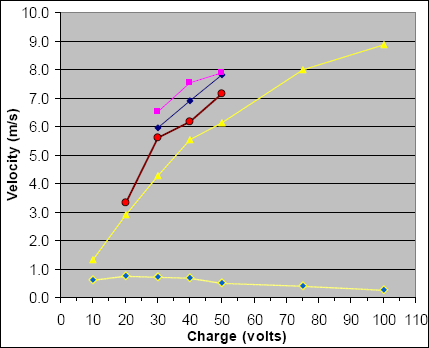# Coil of 97 Turns

This was the first and largest of the coils tested.

Yes, I realize all these numbers and calculations are boring, but this engineering notebook includes all my raw data to permit an independent check of the calculations and conclusions later.

## Coil of 97 Turns

See the Projectiles page for details of these projectiles.

### Measurements

The raw measurements are shown in red in the table below, using horizontal ballistics measurements.

Projectile:

A
C
D
F
Length:   3.5" 2.5" 2" 1.5"
Mass:   4.301 g 2.163 g 1.464 g 0.7345 g
Potential
energy
(joules)

Charge
(volts)
Horiz
Distance
(inches)
Horiz
Distance
(inches)
Horiz
Distance
(inches)
Horiz
Distance
(inches)
0.6 J 10 v 23" - - -
2.4 20 51 58 - -
5.4 30 75 98 104 114
9.6 40 97 108 121 132
15.0 50 107 125 137 138
33.8 75 140 - - -
60.0 100 155 - - -

### Velocity

Using the equations for horizontal ballistics and the raw data, the velocity is calculated for each of the measurements above. The computed velocity is shown in red.

Projectile:

A
C
D
F
Potential
energy
(joules)

Charge
(volts)

Velocity
(m/s)

Velocity
(m/s)

Velocity
(m/s)

Velocity
(m/s)
0.6 J 10 v 1.316 - - -
2.4 20 2.919 3.319 - -

5.4
30 4.292 5.609 5.952 6.524
9.6 40 5.551 6.181 6.925 7.554
15.0 50 6.124 7.154 7.840 7.898
33.8 75 8.012 - - -
60.0 100 8.871 - - -

### Efficiency

Calculate efficiency as shown in red.

Projectile:

A
C
D
F
Potential
energy
(joules)

Charge
(volts)

Efficiency
(%)

Efficiency
(%)

Efficiency
(%)

Efficiency
(%)
0.6 J 10 v 0.6 % - - -
2.4 20 0.8 0.5 - -
5.4 30 0.7 0.6 0.5 0.3
9.6 40 0.7 0.4 0.4 0.2
15.0 50 0.5 0.4 0.3 0.2
33.8 75 0.4 - - -
60.0 100 0.3 - - -

### Graphical Results### Coil Analysis

This coil with 97 turns measured 8 ms half-cycle waveform. The image below indicates peak currents of 350 A at 100v charge.### How Does This Coil Need to Change?

The speeds and efficiencies are very low. We need to shorten the time and increase the peak current.

From the oscilloscope, the half-cycle time is 8 ms, and we desire our next coil to be 25% faster, which means a 6 ms discharge time.

Using a Java RLC simulator, we find the estimated inductance is L = 525 uH for an 8 ms discharge with a 12,000 uF capacitor.

To reduce this to 6 ms, a Java LC Time Simulator finds that inductance should be reduced to a value of 304 uH.

We don’t want to model an iron-core coil in FEM to derive the inductance, so let’s apply the same ratio change to an air-core model. This is much easier to do, and the next bench test will tell us if this method is accurate.

1. We need to reduce inductance L by a factor of (304 uH) / (525 uH) = 0.5790
2. The 97-turn coil in air has inductance L = 186 uH, according to a Java Inductor Sim (using 12 AWG, ID=6mm, OD=69mm, length=16mm).
3. The new coil in air should have L = (186 uH) * (0.5790) = 107.7 uH
4. Using the Java Inductor Sim again, we find an 84-turn coil will have the desired inductance.
5. Therefore, removing 13 turns should result in the desired 6 ms discharge time.
 < Previous Page 30 of 37 Next >